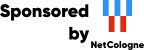## Welcome to the NetCologne GmbH open source mirroring service!

This machine mirrors various open-source projects and has 4 Gbit/s uplink.

If there are any issues or you want another project mirrored, please contact mirror-service -=AT=- netcologne DOT de !

Chapter 6. Statements

## Chapter 6. Statements

This chapter defines the statements of the Comma programming language.

Statement
  Statement `::=` Block_Statement | Null_Statement | Iteration_Statement | Assignment_Statement  Null_Statement `::=` null

A null statement consists of the single reserved word null and has no effect.

## 6.1. Block Statements

Block Statement
  Block_Statement `::=` [ Identifier : ] [ declare { Declarative Item } ] begin Sequence_Of_Statements end [ Identifier ]  Sequence_Of_Statements `::=` Statement ; { Statement ; }

## 6.2. If Statements

If Statement
  If_Statement `::=` if Expression then Sequence_Of_Statements { elsif Expression then Sequence_Of_Statements } [ else Sequence_Of_Statements ] end if

Example 6.1. Examples of if statements:

```      function Fib (X : Natural) return Natural is
begin
if X = 0 then
return 0;
elsif X = 1 then
return 1;
else
return Fib(X - 2) + Fib(X - 1);
end if;
end Fib;
```

## 6.3. Iteration Statements

Iteration Statement
  Iteration_Statement `::=` [ Loop_Tag : ] Loop_Body [ Loop_Tag ]  Loop_Tag `::=` Identifier  Loop_Body `::=` Loop_Statement | While_Statement | For_Statement  Loop_Statement `::=` loop Sequence_Of_Statements end loop  While_Statement `::=` while Expression loop Sequence_Of_Statements end loop  For_Statement `::=` for Identifier in Discrete_Subtype_Definition loop Sequence_Of_Statements end loop

Example 6.2. Examples of iteration statements:

```      type Data is array (Positive range <>) of Integer;

procedure Sort (V : in out Data) is
begin
if V'Length < 2 then
return;
end if;

loop
declare
J      : Integer;
Tmp    : Integer;
Sorted : Boolean := true;
begin
for I in V'First .. V'Last - 1 loop
J := I + 1;
if V(J) < V(I) then
Tmp    := V(I);
V(I)   := V(J);
V(J)   := Tmp;
Sorted := false;
end if;
end loop;

exit when Sorted;
end;
end loop;
end Sort;
```

## 6.4. Assignment Statements

Assignment Statement
  Assignment_Statement `::=` Name := Expression